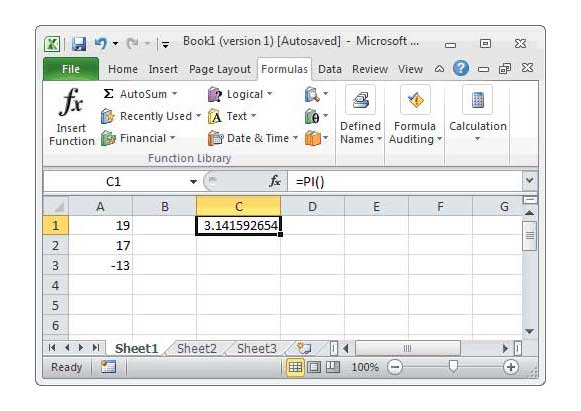# The mathematical constant “pi” represents the ratio between the circumference and the diameter of a circle. If your job requires geometric calculations such as calculating the area of ​​a new office space, you may need to perform operations using the pi number.

You can use pi to calculate the area or volume of a curve, as well as for more complex mathematics, such as trigonometry. Many people consider pi to be 3.14, but Excel stores the exact value of pi up to 15 decimal places.

1. Open your Excel spreadsheet and type ” = ” in an empty cell to label its contents as a mathematical formula.

2. Type the phrase ” () PI ” which is equivalent to ” 3.14159265358979 ” in Excel formula.

3. Type the rest of your formula. For example, to calculate the circumference of a circular area with a radius of 15 cm, the expression

” * 2 * 15 “

Type inside the cell.

4- Press ” Enter ” to execute the formula .

## Tip

Although the PI function stores the pi value in the correct 15 decimal places, Excel rounds up your answer to match the degree of accuracy of the cell. You can increase this degree by using the “Increase Decimal” button in the Number group on the Home tab.